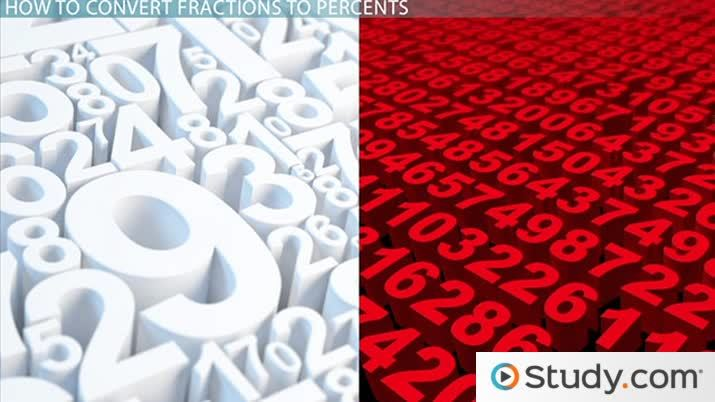# Converting Fractions to Percents

Lesson Transcript
Instructor: Jennifer Beddoe

Jennifer has an MS in Chemistry and a BS in Biological Sciences.

Fractions are numbers that represent parts of a whole and can be converted into percents. Explore the definitions of fraction and percent and learn the methods for converting fractions to percents. Updated: 09/28/2021

## Let's Make a Deal

Have you ever been at the mall shopping for new clothes, and you see a sign - '1/3 off everything on this rack'? Then you turn and see another sign - 'Everything with a red dot is 35% off'. Your budget is tight, so the sale will really help out. But which sale is a better deal? Fortunately, you know how to convert a fraction to a percent, so you can easily tell where to begin looking for the best deal.An error occurred trying to load this video.

Try refreshing the page, or contact customer support.

Coming up next: Ratios & Rates: Definitions & Examples

### You're on a roll. Keep up the good work!

Replay
Your next lesson will play in 10 seconds
• 0:08 Let's Make a Deal
• 0:32 Definitions
• 0:52 How to Convert…
• 3:16 Lesson Summary
Save Save

Want to watch this again later?

Timeline
Autoplay
Autoplay
Speed Speed

## Definitions

A fraction is a number that represents a part of a whole number. Numbers such as 1/2 and 35/72 are fractions.

A percent is a ratio expressed as a fraction of 100. Examples of percents are 25% and 85%

## How to Convert Fractions to Percents

There are two ways to convert a fraction to a percent. Both take a little math skills, but are not difficult at all.

The first method works best with a calculator, but can be done without one. The steps are as follows:

1. Divide the top number by the bottom number.

2. Multiply that result by 100.

For example -

Convert 3/5 to a percent.

3 divided by 5 is 0.6.

0.6 * 100 is 60.

So, 3/5 is 60%.

The second method is to change the fraction so that the denominator is 100. This works because percent means per 100, so if the fraction has 100 as the denominator, the numerator will be the percent.

The steps for converting fractions to percents using this method are:

1. Change your fraction so that the denominator is 100. Remember that whatever you do to change the denominator, you must do the same to the numerator.

2. The new numerator will be your percent.

Let's convert the same example as before, using this new method.

Convert 3/5 to a percent.

To unlock this lesson you must be a Study.com Member.

### Register to view this lesson

Are you a student or a teacher?